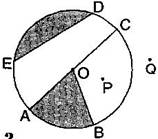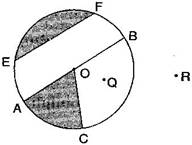### Basic Geometrical Ideas-Solutions Ex-4.6

CBSE Class –VI Mathematics
NCERT Solutions
Chapter 4
Basic Geometrical Ideas (Ex. 4.6)

Question 1. From the figure, identify:(a) The centre of circle.
(c) A diameter.
(d) A chord.
(e) Two points in the interior.
(f) A point in the exterior.
(g) A sector.
(h) A segment.
Answer: (a) O is the centre.
(b) Three radii: OA, OB and OC
(c) A diameter: AC
(d) A chord: ED
(e) Interior points: O, P
(f) Exterior point: Q
(g) A sector: OAB
(h) A segment: ABC
Question 2. (a) Is every diameter of a circle also a chord?
(b) Is every chord of a circle also a diameter?
Answer: (a) Yes, every diameter of a circle is also a chord.
(b) No, every chord of a circle is not a diameter.
Question 3. Draw any circle and mark:
(a) Its centre.